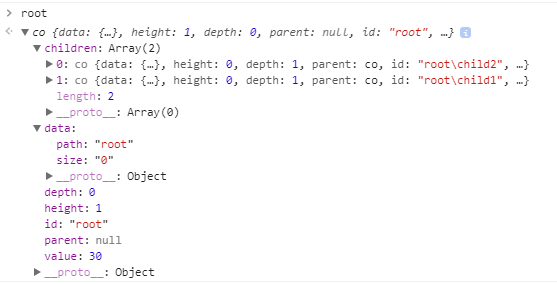# D3 - Hierarchy

tree operation in D3 are done in the hierarchy module.

A tree will be passed to a hierarchical layout such as D3 - Treemap Layout

You must call the function node.sum or node.count before invoking a hierarchical layout that requires node.value, such as d3.treemap.

## Initialisation

### JSON

d3.hierarchy. See example

````d3.hierarchy(data[, childrenFunction])`
```

Default children accessor:

``````function children(d) {
return d.children;
}```
```

### CSV

from csv, see D3 - Stratify (From CSV to tree)

## Properties

A tree have the following properties:

• a children property. An array of nodes of undefined for the leaves
• a depth property (0 for the root to N for the leaves)
• a height property which is the inverse of the depth property (N for the root to 0 for the leaves)
• a id property: the identifier for the node. For leaf nodes, the id may be undefined; otherwise, the id must be unique.
• a parent property. The parent node, or null for the root node.
• a data property : the associated data
• a value property representing the summed value of the node and its descendants (This property can be computed with the sum function. See sum and count).

Null and the empty string are equivalent to undefined.

## Operations

### Visitor

• node.sum(value): Evaluates the specified value function for this node and each descendant in post-order traversal, and returns this node
• node.count(): Computes the number of leaves under this node and assigns it to node.value, and similarly for every descendant of node. If this node is a leaf, its count is one. Returns this node.
• node.sort(compare): Sorts the children of this node, if any, and each of this node’s descendants’ children, in pre-order traversal using the specified compare function, and returns this node.

### Accessors

• node.ancestors(): Returns the array of ancestors nodes, starting with this node, then followed by each parent up to the root.
• node.descendants() : Returns the array of descendant nodes, starting with this node, then followed by each child in topological order.
• node.leaves(): Returns the array of leaf nodes in traversal order; leaves are nodes with no children.
• node.links() : Returns an array of links for this node, where each link is an object that defines source and target properties. The source of each link is the parent node, and the target is a child node.

### Traversal

• node.each(function) Invokes the specified function for node and each descendant in breadth-first order,
• node.eachAfter(function) Invokes the specified function for node and each descendant in post-order traversal, such that a given node is only visited after all of its descendants have already been visited. The specified function is passed the current node.
• node.eachBefore(function): Invokes the specified function for node and each descendant in pre-order traversal, such that a given node is only visited after all of its ancestors have already been visited. The specified function is passed the current node.

### Others

• node.path(target): Returns the shortest path through the hierarchy from this node to the specified target node.
• node.copy(): Return a deep copy of the subtree starting at this node. (The returned deep copy shares the same data, however.) The returned node is the root of a new tree; the returned node’s parent is always null and its depth is always zero.

## Example

``````data = {
"name": "0",
"children": [
{
"name": "1"
},
{
"name": "2",
"children": [
{
"name": "21"
},
{
"name": "22"
}
]
},
{
"name": "3"
},
{
"name": "4",
"children": [
{
"name": "41"
}
]
},
{
"name": "5"
}
]
}
tree = d3.hierarchy(data);

tree.count();
console.log("The leaf count is "+tree.value);
console.log("The leaves are: "+tree.leaves().map( d => d.data.name ));

tree.sum(function(d){ return d.name.length; });
console.log("The sum of all letters in the name is "+tree.value);
console.log("The descendants are: "+tree.descendants().map( d => d.data.name ));

var nodes = [];
tree.each( d => nodes.push(d.data.name) )
console.log("The Each function has visited the tree in this order: "+nodes);
var nodes = [];
tree.eachAfter( d => nodes.push(d.data.name) )
console.log("The eachAfter function has visited the tree in this order: "+nodes);
var nodes = [];
tree.eachBefore( d => nodes.push(d.data.name) )
console.log("The eachBefore function has visited the tree in this order: "+nodes);

console.log(tree.links().map( d => "Source:"+d.source.data.name+", Target: "+d.target.data.name));```
```

## Documentation / Reference

Recommended PagesD3 - Layout Module

Layouts module supply reusable, flexible visualization techniques by generating abstract data structures. The partition layout computes a two-dimensional spatial subdivision of a hierarchical (tree...D3 - Pack

Pack layout to represent a Hierarchy (or a tree). Example authors - Circle packing d3/d3/wiki/Pack-LayoutD3 - Stratify (From CSV to tree)

With a stratify function, you can turn a series of CSV record in a JSON tree object. Since v4 In the stratify object definition, you may change the following properties: the parentId function...D3 - Tree

Data manipulation: layout: Tree - Example: treeD3 - Treemap Layout

treemap in D3 is implemented as a layout. v4. You pass the root node of a tree to the treemap function and it will then add the coordinates of the tile to each node. Create a tree Call tree.sum...